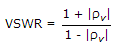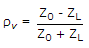Electronics and Communication Engineering - Microwave Communication - Discussion

15.

If VSWR is infinite, the transmission line is terminated in

 [A]. short circuit [B]. complex impedance [C]. open circuit [D]. either (a) or (c)

Explanation:and. Since VSWR = ∞, |rv| = 1. This can happen if ZL = 0 or ZL = ∞, i.e., line is o.c or s.c.